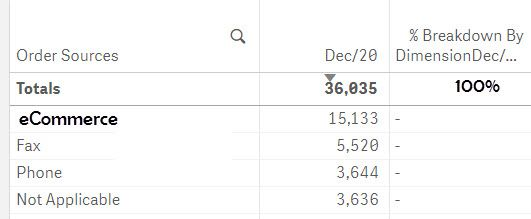# New to Qlik Sense

Discussion board where members can get started with Qlik Sense.

cancel
Showing results for
Did you mean:
HighlightedContributor III

## Calculate breakdown percentage of dimensions

Hello,

I have a straight table that shows the number of orders broken down by order sources. How can I create another measure that shows the breakdown percentage of the total by dimension. For example, if the total number of orders is  36,035 that total is 100% for the month. How can I calculate the % breakdown of that 100% by dimension in a corresponding column?

See below example. I added the 100% for illustrative purposes.1 Solution

Accepted Solutions
HighlightedPartner

## Re: Calculate breakdown percentage of dimensions

Hi,

in your second Measure expression write this and change the  number formatting in %

Count(Orders)/Count(total Orders)

Regards,

Prashant

2 Replies
HighlightedPartner

## Re: Calculate breakdown percentage of dimensions

Hi,

in your second Measure expression write this and change the  number formatting in %

Count(Orders)/Count(total Orders)

Regards,

Prashant

HighlightedPartner

Hi

Try this

Count(Orders)

/

Count({1}Orders)

Hope this helps

Thanks